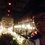# Welcome!

Page 1

Hi everyone!

In this set, we'll be talking about the relationship between the size, shape of a grid and the number of quadrilaterals.

First, we have to know what is a quadrilateral and what is a grid.

Quadrilateral is a polygon with 4 sides and 4 vertices.

## Grids

Grids are shapes made by multiple congruent quadrilaterals aligning together, commonly squares. In this set, we'll all be using grids made with squares. Originally, it should be called grid of squares, but we'll just call it grid for short.GridGrid

All grids have a size, in this set, all sizes of a grid are denoted as $a\times b$ where $a$ is the length of the grid, and $b$ is the height of the grid.

For example, the left diagram above shows a $5\times 5$ grid, $a=b=5$, the right diagram above shows a $4\times 3$ grid, $a=4$, $b=3$.

The variables $a$ and $b$ will be frequently used in the following pages.

This is one part of Grids and Quadrilaterals.Note by Kenneth Tan
5 years, 6 months ago

This discussion board is a place to discuss our Daily Challenges and the math and science related to those challenges. Explanations are more than just a solution — they should explain the steps and thinking strategies that you used to obtain the solution. Comments should further the discussion of math and science.

When posting on Brilliant:

• Use the emojis to react to an explanation, whether you're congratulating a job well done , or just really confused .
• Ask specific questions about the challenge or the steps in somebody's explanation. Well-posed questions can add a lot to the discussion, but posting "I don't understand!" doesn't help anyone.
• Try to contribute something new to the discussion, whether it is an extension, generalization or other idea related to the challenge.

MarkdownAppears as
*italics* or _italics_ italics
**bold** or __bold__ bold
- bulleted- list
• bulleted
• list
1. numbered2. list
1. numbered
2. list
Note: you must add a full line of space before and after lists for them to show up correctly
paragraph 1paragraph 2

paragraph 1

paragraph 2

[example link](https://brilliant.org)example link
> This is a quote
This is a quote
    # I indented these lines
# 4 spaces, and now they show
# up as a code block.

print "hello world"
# I indented these lines
# 4 spaces, and now they show
# up as a code block.

print "hello world"
MathAppears as
Remember to wrap math in $$ ... $$ or $ ... $ to ensure proper formatting.
2 \times 3 $2 \times 3$
2^{34} $2^{34}$
a_{i-1} $a_{i-1}$
\frac{2}{3} $\frac{2}{3}$
\sqrt{2} $\sqrt{2}$
\sum_{i=1}^3 $\sum_{i=1}^3$
\sin \theta $\sin \theta$
\boxed{123} $\boxed{123}$

Sort by:

Nice problems.....Sooooooooo easy!!!

- 5 years, 6 months ago